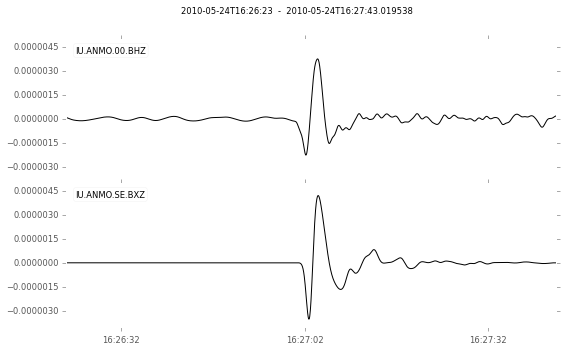# Using Syngine with ObsPy¶

This is a quick tutorial on how to use the Syngine service with ObsPy.

In :
# First a bit of setup to make the plots appear in the
# notebook and make them look a bit nicer.
%matplotlib inline
import matplotlib.pyplot as plt
plt.style.use("ggplot")


In :
import obspy
from obspy.fdsn import Client

# Create a client instance connected to IRIS.
c = Client("IRIS")


This example deals with a deep event so we expect a very clear first P arrival and weak surface waves.

#### (Optional) Acquire Event Information¶

In :
cat = c.get_events(starttime=obspy.UTCDateTime(2010, 5, 24, 16),
endtime=obspy.UTCDateTime(2010, 5, 24, 17),
minmagnitude=6.4)
print("Event depth in km:", cat.origins.depth / 1000.0)
cat.plot(projection="ortho");

Event depth in km: 582.1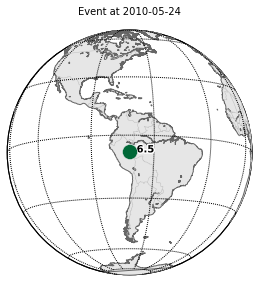We will now use the FDSN client to download the observed data as well as the instrument response.

In :
# The attach_response argument will cause a second request to the
# station service which fetches the instrument response.
st = c.get_waveforms(network="IU", station="ANMO", location="00", channel="BHZ",
starttime=obspy.UTCDateTime(2010, 5, 24, 16, 18, 28),
endtime=obspy.UTCDateTime(2010, 5, 24, 17, 18, 28),
attach_response=True)
st.plot()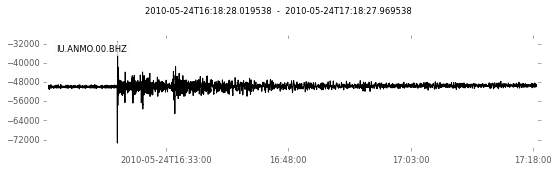The next step is to convert it to physical units. The pre filter here is very wide but it is a very good station so that is ok here.

In :
st.remove_response(output="DISP", pre_filt=(0.005, 0.01, 4, 8))
st.plot()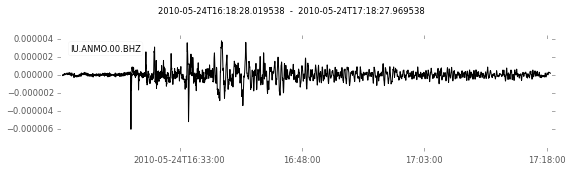In :
st_synth = obspy.read("http://service.iris.edu/irisws/syngine/1/query?"
"network=IU&station=ANMO&components=Z&"
"eventid=GCMT:C201005241618A&dt=0.05&"
"units=displacement&"
"model=iasp91_2s&label=Tutorial&format=miniseed")
st_synth.plot()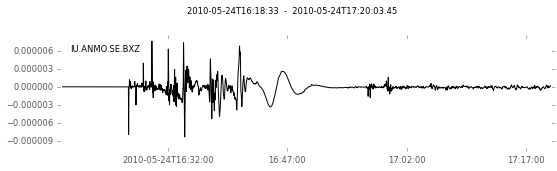#### (Optional) Calculate Theoretical Arrival Time¶

In :
from obspy.taup import TauPyModel

m = TauPyModel("IASP91")
arr = m.get_travel_times(
source_depth_in_km=582.1, distance_in_degree=54.16,
phase_list=["P"])
print(arr)

1 arrivals
P phase arrival at 511.424 seconds


#### Plot P Arrival¶

In the generated plot the observed data is on the top, and the synthetic on the bottom.

In :
# Combine into a single Stream object.
st_all = st.copy() + st_synth.copy()

# Filter to a period band from 1 to 10 seconds to highlight
# the P phase and force the same band limit on both.
st_all.detrend("demean")
st_all.taper(0.05)
st_all.filter("bandpass", freqmin=0.1, freqmax=1.0, corners=3)

# Cut window around the P arrival
starttime = st_synth.stats.starttime + 470
endtime = st_synth.stats.starttime + 550
st_all.trim(starttime, endtime)

st_all.plot()